## 2.2.4 Numerics

As shown in Section 2.2.3 the grid sizes rise dramatically in three dimensions. The discretization (see Section 2.4) produces coupling between grid points where only neighboring points of a certain point are of interest which are directly connected to this point by lines - the grid lines. Compared with the large matrix sizes this coupling is very small resulting in very sparsely filled equation systems.  The number of connections to other points depends on the grid and is normally between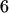to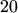for the three-dimensional case.

To solve the nonlinear semiconductor equations the Newton scheme is applied. Therefore, for each iteration a system matrix (Jacobian) of a linear system must be created. In order to keep the memory and time consumptions low it is mandatory to store the system matrix in a so called sparse matrix. In Minimos-NT the MCSR format  is used. MCSR consists of two arrays. In the first array the column indices of the off-diagonal entries are stored row by row. To quickly find the beginning of a row the indices are preceeded by an index table containing the start indices of each row. The second array stores the main diagonal entries followed by all non-zero off-diagonal entries.

Thus, all numerical algorithms have to work on sparse matrices. The solvers are called sparse linear solvers. In order to achieve diagonal dominant matrices some equations known before are removed from the system to be solved afterwards by a Gaussian algorithm which is especially true for boundary conditions .

For two-dimensional simulations both sparse direct solvers and sparse iterative solvers are commonly used. Sparse direct solvers are based on Gaussian elimination approach. They are very reliable and produce a solution even for ill-conditioned matrices where iterative solvers fail to converge. The disadvantages of direct solvers are the high memory consumption and the high solving time. The memory consumption rises superlinearly with the matrix sizes which is not affordable for large three-dimensional grids. The memory consumption heavily depends on the structure of the matrix as additional elements occur in the matrix during factorization which are termed fill-in. Several algorithms exist, e.g., reverse Cuthill-McKee , to reduce the bandwidth of the matrix in order to achieve low fill-in. Furthermore the time to solve the linear system is much higher than for iterative solvers and rises with an order of magnitude of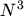where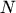is the number of rows of the matrix.

Iterative solvers used in device simulation are based on the conjugate gradient method (CG)  which strongly relies on good preconditioning algorithms which are responsible for the condition of the linear system and thus for the convergence of the solving process.

The memory consumption of iterative solvers is predictable low. Only a fixed amount of memory is used and for each iteration a fixed amount of work has to be done. Iterative solvers usually are much faster than direct solvers. The iteration process is terminated if a specified accuracy is reached. For that reason the solution has a predictable low error if the system converges. The procedure is terminated also if a maximum of allowed iterations is exceeded. In that case the system cannot be solved and either the preconditioning parameters are changed or the direct solver has to be used. The number of iterations strongly depends on the numerical values of the linear system. So the system matrix is usually preconditioned. In case of Minimos-NT the ILU is used which requires a proper scaling of the matrix. The scaling enables the preconditioner to decide whether an entry is skipped or not. Thereby the main diagonal elements are scaled to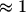and the off-diagonal entries should become as low as possible. In contrary to direct solvers no error propagation is possible for iterative solvers.

In  time comparisons of a symmetric iterative solver (preconditioned conjugate gradient solver ICCG) and a direct solver (Gaussian solver) are shown. For a matrix size of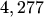ICCG requiresand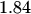MBytes whereas the Gaussian solver requires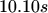and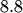MBytes of memory. For a higher matrix size of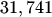ICCG requires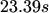and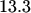MBytes. However, the requirements of the Gaussian solver rise superlinearly to a solving time of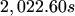and a memory consumption of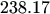MBytes.

Therefore, direct solvers cannot be used for three-dimensional simulations [89,90]. Because non-symmetric equation systems have to be solved in Minimos-NT the iterative solver Bi-CGSTAB  is used in combination with an ILU preconditioner. Another improved version of this solver is also available, which is capable to calculate systems with complex numbers and is therefore well suited for AC-small signal analyzes, too.

Robert Klima 2003-02-06